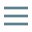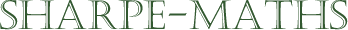Previous topic:
Multiplying fractions
Current topic:
Dividing fractions
Next topic:
Multiplying & dividing mixed numbers

### Dividing fractions

There's an old joke, I am rather fond of, which goes "How do you get down off an elephant?", to which the answer is "You don't! You get down off a duck."

This is borderline irrelevant but included here a) because I like it and b) because it uses a humourous device based on the idea that to do something, it is often better to begin somewhere other than where you are at present. Like the person asking for directions, who gets the reply, "Well, I wouldn't start from here."

Here's a more relevant question: "How do you divide fractions?" and the answer is "You don't! You turn the division into a multiplication problem and then solve that."

There are, in fact several ways to divide fractions, but by miles the simplest is to rewrite the division problem as a multiplication problem.

To do this, we need to understand the idea of a reciprocal in mathematics.

Put simply, the reciprocal of a number is that number which multiplies by the original number to give $1$. The reciprocal is the multiplicative inverse of the original number.

Here is an example $\frac{2}{5} \times \frac{5}{2} = 1$. In this statement we can see that these fractions will multiply together to make $1$. The calculation below shows how the cancellation would work.

\begin{aligned} & \frac{2}{5} \times \frac{5}{2}\\ &= \frac{\cancelto{1}{2}}{\cancelto{1}{5}} \times \frac{\cancelto{1}{5}}{\cancelto{1}{2}}\\ &=1 \end{aligned}

Now let us look at a more generalised version.

\begin{aligned} & \frac{a}{b} \times \frac{b}{a}\\ &= \frac{\cancelto{1}{a}}{\cancelto{1}{b}} \times \frac{\cancelto{1}{b}}{\cancelto{1}{a}}\\ &=1 \end{aligned}

What you should notice is that the reciprocal of any fraction is the same fraction turned upside down

This means that dividing by a fraction does exactly the same thing as multiplying by the fraction's reciprocal. So we end up with something like the following.

\begin{aligned} & \frac{3}{5} \div \frac{4}{5}\\ &= \frac{3}{5} \times \frac{5}{4}\\ &= \frac{3}{\cancelto{1}{5}} \times \frac{\cancelto{1}{5}}{4}\\ &=\frac{3}{4} \end{aligned}